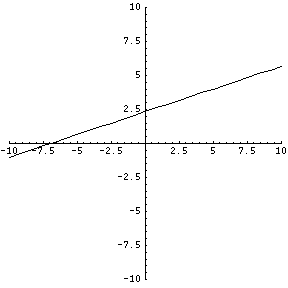# Graphing a Line

### Question:

Hello,
I have a math problem I need some help with. The line goes through (-1,2) and is perpendicular to 3x +Y = 5. Can you show this on a graph in addition, show me the steps please.
Thank you

What two things do you need for a line? A point and a slope. The problem tells us that the line goes through the point (-1,2), so all we need is a slope. Since our line will be perpendicular to 3x+y=5, its slope will be the "negative reciprocal" of that line's slope. That's just something you have to learn:

Perpedicular slopes are inverse (or negative)reciprocals.

Now, what is the slope of that line 3x+y=5? Let's write it in a clearer format -- the y=mx+b format -- and then simplify:

$$3x + y = 5$$ $$y = 5 - 3x$$ $$y = -3x + 5$$

Now you can tell the slope is -3. The slope for our PERPENDICULAR line is the inverse/negative reciprocal of that. First we put a negative sign in front of it: --3. Since two negatives makes a positive, we have +3. Now, take the reciprocal. That's 1/3. Therefore the slope of our new line is 1/3 because 1/3 is the negative reciprocal of the original -3.

We have a point (-1,2) and a slope (1/3). If you want the equation for this line, I would certainly recommend using point-slope format:

$$y-y_1 = m(x-x_1)$$ $$y-2 = \frac{1}{3}(x+1)$$ $$y-2 = \frac{1}{3}(x+1)$$

And of course you can rewrite that in y=mx+b format if you want to rearrange it.

Now I know you wanted a graph. First, I'm going to let you know how to do it on your own. The line passes through (-1,2) and has a slope of 1/3. That means for every 1 unit you go up, you go 3 units to the right. Can you draw this graph now? Here's what it should look like: